# Density Matrix

Also found in: Acronyms, Wikipedia.

## Density matrix

A matrix which is constructed as the most general statistical description of the states of a many-particle quantum-mechanical system. The state of a quantum system is described by a normalized wave function ψ(x, t) [where x stands for all coordinates of the system, and t for the time], which satisfies the Schrödinger equation (1), where H is

(1)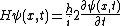the hamiltonian of the system, and &hstrok; is Planck's constant divided by 2π. Furthermore, ψ(x, t) may be expanded in terms of a complete orthonormal set {&phiv;(x)}, as in Eq. (2). Then, the
(2)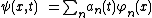(3)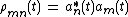density matrix is defined by Eq. (3), and this density matrix describes a pure state. Examples of pure states are a beam of polarized electrons and the photons in a coherent beam emitted from a laser. See Laser, Quantum mechanics

In quantum statistics, one deals with an ensemble of N systems which have the same hamiltonian. If the αth member of the ensemble is in the state ψα in Eq. (4), the density matrix is defined as the ensemble average, Eq. (5).

(4)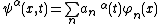(5)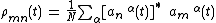In general, this density matrix describes a mixed state, for example, a beam of unpolarized electrons or the photons emitted from an incoherent source such as an incandescent lamp. The pure state is a special case of the mixed state when all members of the ensemble are in the same state. See Statistical mechanics

McGraw-Hill Concise Encyclopedia of Physics. © 2002 by The McGraw-Hill Companies, Inc.
The following article is from The Great Soviet Encyclopedia (1979). It might be outdated or ideologically biased.

## Density Matrix

an operator by means of which it is possible to calculate the average value of any physical quantity in quantum statistical mechanics and, in particular, in quantum mechanics. A density matrix describes a system’s state based on an incomplete set (incomplete in terms of quantum mechanics) of data on the system (seeMIXED STATE).

The Great Soviet Encyclopedia, 3rd Edition (1970-1979). © 2010 The Gale Group, Inc. All rights reserved.

## density matrix

[′den·səd·ē ′mā·triks]
(quantum mechanics)
A matrix ρ mn describing an ensemble of quantum-mechanical systems in a representation based on an orthonormal set of functions φ n; for any operator G with representation Gmn, the ensemble average of the expectation value of G is the trace of ρ G.
McGraw-Hill Dictionary of Scientific & Technical Terms, 6E, Copyright © 2003 by The McGraw-Hill Companies, Inc.
References in periodicals archive ?
The theoretical solutions for the correlation function matrix and the spectral density matrix are discussed and it is pointed out that care should be taken in order to use the second order information in a form that in fact represents free decays of the system.
and the off-diagonal density matrix elements relevant to the electric- and magnetic-dipole allowed transitions are of the form
Thus Unruh's "which way" statement when both paths of the interferometer are unimpeded is equivalent to the statement that the density matrix of the photons at the detectors is a mixed one.
Intragroup densities, [d.sub.ii], are found on the main diagonal of a density matrix and are calculated by the formula [d.sub.ii] = N/[n.sub.1] ([n.sub.11] - 1).
On the one hand, the Schrodinger equation with modification (non-linear Schrodinger equation) and/or the density matrix operator have been some primary mathematical tools to assess collapse.
All information about the system is involved in the total density matrix (9).
Given that [N.sub.s] [greater than or equal to] [N.sub.0], the averaged spectral density matrix estimator follows the central complex [N.sub.0] -variate Wishart distribution with [N.sub.s] degrees of freedom.
The big advantage of FLOUP is that it places a calculable point at which the density matrix becomes a classical probability, as will be discussed in Section 2.
The expectation values of the operators on the right-hand side correspond to the components of the spin-resolved one-particle reduced density matrix
From the {[c.sub.n[mu]]} coefficients, we obtain the ground state density matrix
Also, matrix D, which is known as the force density matrix (FDM) of the tensegrity, relates the nodal coordinates and the forces which are acting on the structure and is given by

Site: Follow: Share:
Open / Close